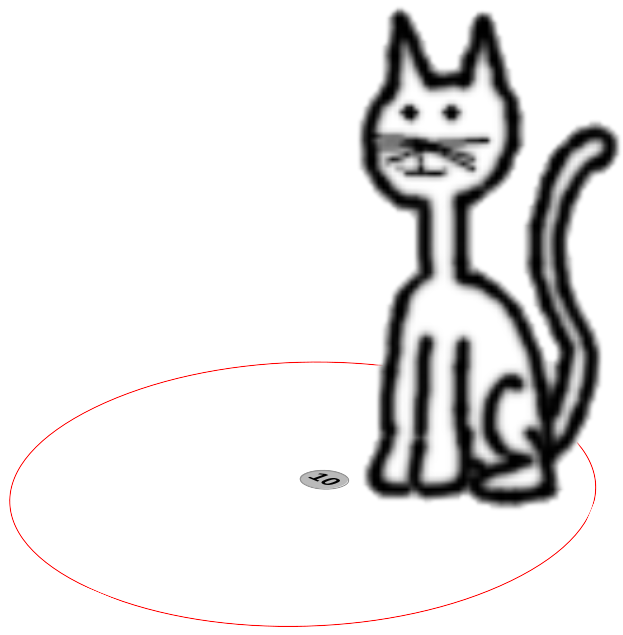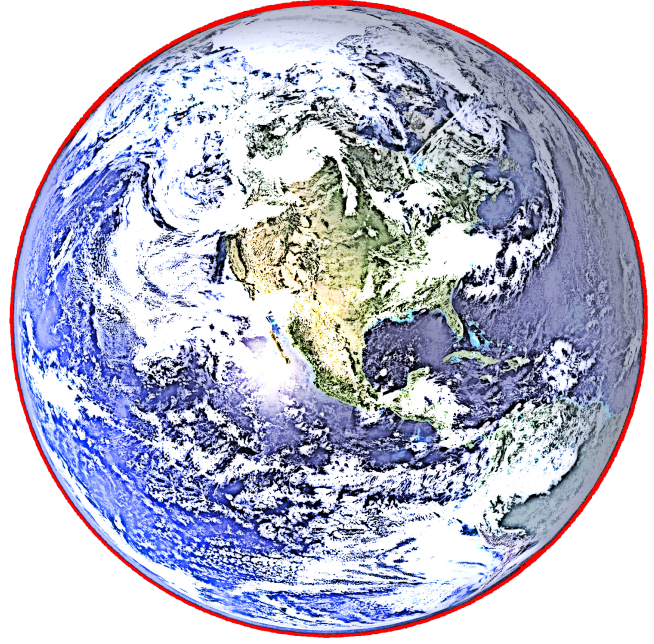Imagine the following context. We put a 10 cent coin with a 1cm radius on the floor. Then we take a string and go around the coin, for that we will need 2.π cm of string, approximately 6 cm.So we decided to make a circle of sufficient size so that a cat could pass between the coin and the string. For this, we need to increase the amount of string with which we will go around the coin, keeping the coin in the center. Assuming it is not a fat cat, then 10 cm should fit between the coin and the string. Thus, we will need a circle with a radius of 11 cm (10 for the cat, 1 for the coin). The total string will now be something close to 69 cm. That is, the amount of string that we need to add to our cat was approximately 63 cm.Now let’s go to an interesting case, imagine that the Earth’s gravity was turned off for a moment, and then we put a string around it (we assume its radius is exactly 6.371 km). The string we need to make this complete contour around the Earth will be 40.030 kilometers, 173 meters and 59 centimeters.In this situation, the string is fully stretched close to the earth’s surface. So the question arises, how much more string will we need to increase the radius of this circumference by 10 centimeters, so that a cat in any position on the planet that meets this string, can pass between the Earth’s surface and the string?

The first time this problem is described to us, we imagine that to increase the circumference of a proportion like the planet, from which it took more than 40.000 km of string to get around it, we would need a very large amount of string as well. But oddly enough, the answer is 63 cm. With just another 63 cm of string, we took the string that was fully stretched before the planet’s surface, and thus increased its circumference giving the space between it and the planet by 10 cm. Enough for a cat to pass.

The reasoning error that leads us to imagine that we will need an enormous amount of string for this, is due to the difficulty in realizing that the equation of the circumference length is given by

If we go around the Earth, we will have:

2.π. (Earth-ray).

In case we go around the Earth and give another 10 cm to this circle, we will have: